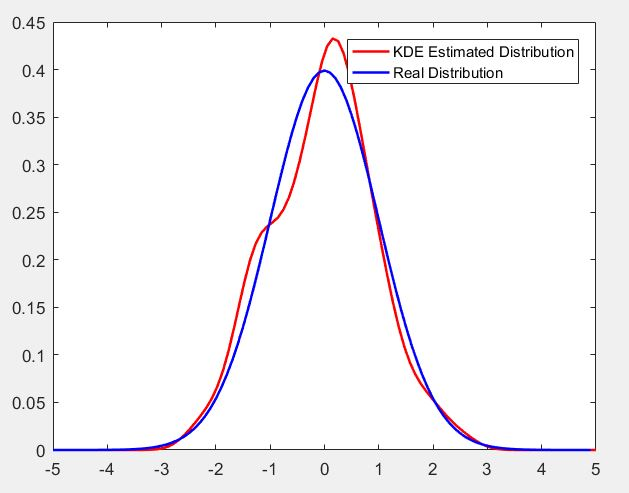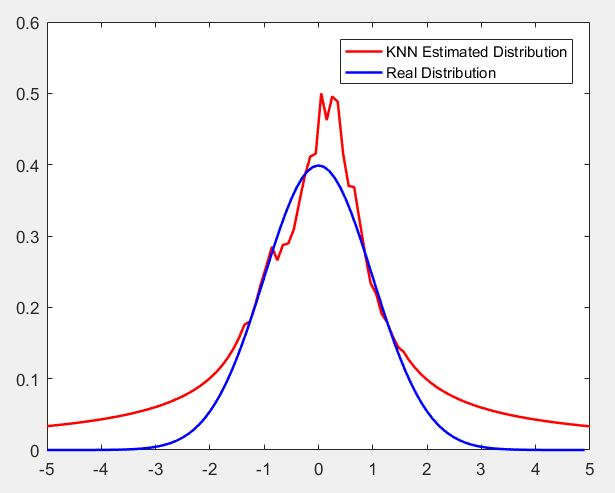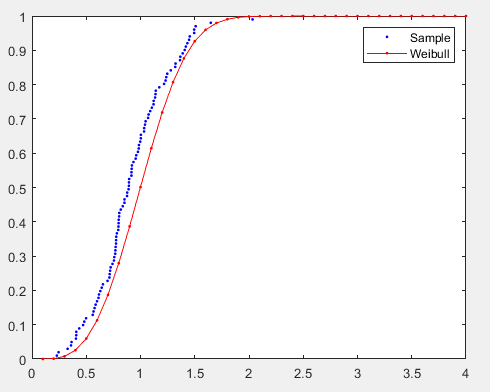• 参数估计MATLAB实现
千次阅读
2021-04-22 06:25:43

《参数估计的MATLAB实现》由会员分享，可在线阅读，更多相关《参数估计的MATLAB实现(17页珍藏版)》请在人人文库网上搜索。

1、1,参数估计MATLAB实现,点估计,区间估计,2,点估计,区间估计,矩估计,最大似然估计,参数估计,点估计,参数估计主要内容,3,点估计,Matlab统计工具箱给出了常用概率分布中参数的点估计(采用最大似然估计法)与区间估计,另外还提供了部分分布的对数似然函数的计算功能.由于点估计中的矩估计法的实质是求与未知参数相应的样本的各阶矩,可根据需要选择合适的矩函数进行点估计.,4,矩估计的MATLAB实现,B2,所以总体X均值及方差的矩估计可由下MATLAB命令实现：,mu_ju=mean(X)sigma2_ju=moment(X,2),为总体样本,求未知参数的矩估计.,5,x=232.50,23。

2、2.48,232.15,232.52,232.53,232.30,.232.48,232.05,232.45,232.60,232.47,232.30;,mu_ju=mean(X)sigma2_ju=moment(X,2),例：来自某总体X的样本值如下：232.50,232.48,232.15,232.52,232.53,232.30,232.48,232.05,232.45,232.60,232.47,232.30求X的均值与方差的矩估计,矩估计的MATLAB实现,6,MLE,通用命令mle()格式：输出参数项=mle(分布函数名,X,alpha,N),说明：分布函数名有：bino(二项)、。

3、geo(几何)、hyge(超几何)、poiss(泊松),uinf(均匀)、unid(离散均匀)、exp(指数)、norm(正态),t(T分布)、f(F分布)、beta(贝塔)、gam(伽吗)；N当为二项分布时需要，其他没有。,7,MLE,例设从一大批产品中抽取100个产品，经检验知有60个一级品，求这批产品的一级品率的极大似然估计.,clear;alpha=0.05;N=100;X=60;mle(bino,X,alpha,N),8,MLE,例设从一大批产品中抽取100个产品，经检验知有60个一级品，求这批产品的一级品率(置信度95%)。,clear;alpha=0.05;N=100;X=60;。

4、Ph,Pc=mle(bino,X,alpha,N),Ph=0.6000Pc=0.4972,0.6967,95%置信区间,9,用matlab产生随机数,通用函数,y=random(分布的英文名,A1,A2,A3,m,n),表示生成m行n列的mn个参数为(A1,A2,A3)的该分布的随机数,例：R=random(Normal,0,1,2,4),例R=random(Poiss,3,100,1),生成参数为3，100个服从Poisson分布的随机数,生成参数为2行4列服从标准正态分布的随机数,10,用matlab产生随机数,专用函数,1、R=normrnd(mu,sigma,m,n),生成参数为N,P。

5、的m行n列的二项分布随机数,例R=normrnd(0,1,3,2),2、R=unifrnd(a,b,m,n),生成a,b上的m行n列的泊松分布随机数,例unifrnd(0,1,1,6),11,生成随机数专用函数表,12,区间估计的MATLAB实现,如果已经知道了一组数据来自正态分布总体，但是不知道正态分布总体的参数。我们可以利用normfit()命令来完成对总体参数的点估计和区间估计，格式为mu,sig,muci,sigci=normfit(x,alpha),13,mu,sig,muci,sigci=normfit(x,alpha),Muci、sigci分别为分布参数、的区间估计。,x为向量或。

6、者矩阵，为矩阵时是针对矩阵的每一个列向量进行运算的。,alpha为给出的显著水平(即置信度，缺省时默认，置信度为95),mu、sig分别为分布参数、的点估计值。,区间估计的MATLAB实现,14,例从某超市的货架上随机抽取9包0.5千克装的食糖，实测其重量分别为(单位：千克)：0.497，0.506，0.518，0.524，0.488，0.510，0.510，0.515，0.512，从长期的实践中知道，该品牌的食糖重量服从正态分布。根据数据对总体的均值及标准差进行点估计和区间估计。,x=0.497,0.506,0.518,0.524,0.488,0.510,0.510,0.515,0.512;。

7、alpha=0.05;mu,sig,muci,sigci=normfit(x,alpha),区间估计的MATLAB实现,15,a、b、aci、bci分别是均匀分布中参数a,b的点估计及区间估计值。,其它常用分布参数区间估计的命令,lam,lamci=poissfit(x,alpha)泊松分布的估计函数,lam、lamci分别是泊松分布中参数的点估计及区间估计值。,a,b,aci,bci=unifit(x,alpha)均匀分布的估计函数,16,p、pci分别是二项分布中参数的点估计及区间估计值。,lam,lamci=expfit(x,alpha)指数分布的估计函数,lam、lamci分别是指数分布中参数的点估计及区间估计值,p,pci=binofit(x,alpha)二项分布的估计函数,其它常用分布参数估计的命令还有：,17,例调查某电话呼叫台的服务情况发现:在随机抽取的200个呼叫中，有40%需要附加服务(如转换分机等)，以p表示需附加服务的比例，求出p的置信度为0.95的置信区间。,R=200*0.4;n=200;alpha=0.05;phat,pci=binofit(R,n,alpha),phat=0.4000，pci=0.33150.4715。

更多相关内容
• 概率密度函数非参数估计matlab代码matLearn matLearn：Matlab中的机器学习算法实现 免责声明： 该软件包是2014年秋季我们在机器学习课程中集体努力的结果，CPSC 540由UBC的Mark Schmid博士教授。 那时，还没有任何...
• 参数估计 function [mu, sigma] = Bayesian_parameter_est(train_patterns, train_targets, sigma)贝叶斯
• 沪深股市日收益率的二元copula模型，copula函数的选取 参数估计 深证和上证的尾部相关性
• 概率密度函数非参数估计matlab代码使用Python进行均值平移分割 执行均值平移分割以跟踪图像序列上的对象。 均值漂移细分实施者：Agam Deep Arora（50169805）和Debika Dutt（50170009）提交日期：15年12月14日2.文献...
• 概率密度函数非参数估计matlab代码代码-Matlab 2017a / Python 3.7-MSAL（多标准优化主动学习）算法 主动学习选择最关键的实例，并通过与Oracle的交互来获取它们的标签。 选择信息量大或代表性的未标记实例可能会...
• copula_copula_copula参数估计matlab_收益模型_尾部相关.zip
• 遗忘因子递推最小二乘参数估计，用于识别系统，MATLAB程序 遗忘因子递推最小二乘参数估计，用于识别系统，MATLAB程序
• 参数估计matlab函数表 参数估计函数表 函数名 调用形式 函数说明 binofit PHAT= binofit(X, N) [PHAT, PCI] = binofit(X,N) [PHAT, PCI]= binofit (X, N, ALPHA) 二项分布的概率的最大似然估计 置信度为 95%的参数...参数估计matlab函数表

参数估计函数表 函数名 调用形式 函数说明 binofit PHAT= binofit(X, N) [PHAT, PCI] = binofit(X,N) [PHAT, PCI]= binofit (X, N, ALPHA) 二项分布的概率的最大似然估计 置信度为 95%的参数估计和置信区间 返回水平 α 的参数估计和置信区间 poissfit Lambdahat=poissfit(X) [Lambdahat, Lambdaci] = poissfit(X) [Lambdahat, Lambdaci]= poissfit (X, ALPHA) 泊松分布的参数的最大似然估计 置信度为 95%的参数估计和置信区间 返回水平 α 的 λ 参数和置信区间 normfit [muhat,sigmahat,muci,sigmaci] =normfit(X) [muhat,sigmahat,muci,sigmaci] = normfit(X, ALPHA) 正态分布的最大似然估计，置信度为 95% 的置信区间 返回水平 α 的期望、方差值和置信区间 betafit PHAT =betafit (X) [PHAT, PCI]= betafit (X, ALPHA) 返回 β 分布参数 a 和 b 的最大似然估计 返回最大似然估计值和水平 α 的置信区间 unifit [ahat,bhat] = unifit(X) [ahat,bhat,ACI,BCI] = unifit(X) [ahat,bhat,ACI,BCI]=unifit(X, ALPHA) 均匀分布参数的最大似然估计 置信度为 95%的参数估计和置信区间 水平 α 的参数估计和置信区间 expfit muhat =expfit(X) [muhat,muci] = expfit(X) [muhat,muci] = expfit(X,alpha) 指数分布参数的最大似然估计 置信度为 95%的参数估计和置信区间 水平 α 的参数估计和置信区间 gamfit phat =gamfit(X) [phat,pci] = gamfit(X) [phat,pci] = gamfit(X,alpha) γ 分布参数的最大似然估计 置信度为 95%的参数估计和置信区间 最大似然估计值和水平 α 的置信区间 weibfit phat = weibfit(X) [phat,pci] = weibfit(X) [phat,pci] = weibfit(X,alpha) 韦伯分布参数的最大似然估计 置信度为 95%的参数估计和置信区间 返回水平 α 的参数估计及其区间估计 Mle phat = mle( dist ,data) [phat,pci] = mle( dist ,data) 分布函数名为 dist 的最大似然估计 置信度为 95%的参数估计和置信区间 [phat,pci] = mle( dist ,data,alpha) [phat,pci] = mle( dist ,data,alpha,p1) 返回水平 α 的最大似然估计值和置信区间 仅用于二项分布，pl 为试验总次数说明 各函数返回已给数据向量 X 的参数最大似然估计值和置信度为(1- α)×100%的置信区间。α 的默认值为 0.05，即置信度为 95% 。

展开全文• 提供了AR模型参数估计完整matlab代码，与这篇文章中的原理部分组合https://blog.csdn.net/weixin_44846910/article/details/107311415，就是一篇完整的实验报告。 编程实现 Levinson- Durbin递推算法。 1.使用AR模型...
• 本资源为利用递推最小二乘算法对永磁同步电机的所有四个参数（定子电阻，转子磁链，d轴和q轴电感进行在线估计的仿真模型和有逆变器死区补偿的参数辨识模型
• 跳频信号参数估计,跳频信号参数估计 Matlab代码,matlab源码.zip
• 概率密度函数非参数估计matlab代码人工智能笔记 我所了解的有关人工智能/数据科学/机器学习/统计建模/模式识别/您想要称呼本笔记内容的一切。 所有这些之间的界线都非常模糊，但是它们都试图回答相同的问题：“我们...
• 主要是关于logistic的三种求参数的算法：Yule算法，Rhodes算法，Nair算法matlab算法的实现。logistic算法
• 核密度非参数估计matlab代码Non_Parametric_Kernel_Density_Estimation 我们建议使用基于核密度估计 (KDE) 的方法进行分类。 这种非参数方法本质上以一种有原则的方式为每个类提供了成员资格的可能性。 该实现用于...
• copula_copula_copula参数估计matlab_收益模型_尾部相关_源码.rar
• 机器学习（二）非参数估计matlab例程 2018/2/19 by ChenjingDing 问题描述： 分别使用K近邻和核函数的方法。为输入样本xˆx^\widehat{x}估计概率密度函数。xˆx^\widehat{x} = linspace(-5, 5, 100); 假设...

## 机器学习（二）非参数估计matlab例程

2018/2/19
by ChenjingDing

问题描述：
分别使用K近邻和核函数的方法。为输入样本 xˆ x ^ $\widehat{x}$估计概率密度函数。 xˆ x ^ $\widehat{x}$ = linspace(-5, 5, 100);
假设 xtrai x t r a i $x_{trai}$ = random(‘norm’, 0, 1, 1, 100)服从高斯分布，则估计出的概率密度也应该服从高斯分布。

apply.m

close all;
clc;

%get fixed K of knn and fixed h of kde
p = parameters();

disp('Question: Kernel/K-Nearest Neighborhood Density Estimators');

% Produce the random samples
samples = random('norm', 0, 1, 1, 100);

% Compute the original normal distribution
realDensity = gauss1D(0, 1, 100, 5);

% Estimate the probability density using the KDE
estDensity = kde(samples, p.h);

% plot results
figure;
plot(estDensity(1, :), estDensity(2, :), 'r', 'LineWidth', 1.5);
hold on;
plot(realDensity(1, :), realDensity(2, :), 'b', 'LineWidth', 1.5);
legend('KDE Estimated Distribution', 'Real Distribution');
hold off;

% Estimate the probability density using KNN
estDensity = knn(samples, p.k);

% Plot the distributions
figure;
plot(estDensity(1, :), estDensity(2, :), 'r', 'LineWidth', 1.5);
hold on;
plot(realDensity(1, :), realDensity(2, :), 'b', 'LineWidth', 1.5);
legend('KNN Estimated Distribution', 'Real Distribution');
hold off;



knn.m

function estDensity = knn(samples, k)
% compute density estimation from samples with KNN
% Input
%  samples    : DxN matrix of data points
%  k          : number of neighbors
% Output
%  estDensity : estimated density in the range of [-5, 5]

% Compute the number of the samples created
N = length(samples);

% Create a linearly spaced vector
pos = linspace(-5, 5, 100);

% Create two big matrices to avoid for loops
x = repmat(pos, N, 1);
samples = repmat(samples', 1, length(pos));

% Sort the distances so that we can choose the k-th point
dists = sort(abs(x-samples), 1);

% Estimate the probability density using the k-NN density estimation
% dists(k, :) = V/2;
res = (k/(2*N)) ./ dists(k, :);

% Form the output variable
estDensity = [pos; res];

end



kde.m

function estDensity = kde(samples, h)
% compute density estimation from samples with KDE
% Input
%  samples    : DxN matrix of data points
%  h          : (half) window size/radius of kernel
% Output
%  estDensity : estimated density in the range of [-5,5]

% Compute the number of samples created
N = length(samples);

% Create a linearly spaced vector
pos = linspace(-5, 5, 100);

% Create two big matrices to avoid for loops
x = repmat(pos, N, 1);
samples = repmat(samples', 1, length(pos));

% Estimate the density from the samples using a kernel density estimator
% 参考机器学习（二）非参数估计核函数法 高斯函数一例
res = sum(exp(-(x-samples).^2./(2*h^2)), 1) ./ (sqrt(2*pi)*h*N);

% Form the output variable
estDensity = [pos; res];

end



gauss1D.m

function [realDensity] = gauss1D(m, v, N, w)

pos = (-w:(2*w/N):w-w/N);
meanV = repmat(m,N,1)';

aux = pos - meanV;
insE = (aux.*aux)./(v^2)*(-0.5);
norm = 1/(v*sqrt(2*pi));

res = norm.*exp(insE);

realDensity = [pos;res];

end



parameter.m

function p = parameters()
p.k = 30; %knn neighbors
end

结果如下：图8 核函数估计概率密度结果（红色曲线为估计值，蓝色曲线为理想值）图9 K近邻法估计概率密度结果（红色曲线为估计值，蓝色曲线为理想值）

展开全文• 【代码特点】：参数化编程（参数可方便修改）、思路步骤清晰、注释明细。 【适合对象】：信号处理、雷达专业学生。 【乱码问题】：文件打开如果出现中文注释乱码的情况，就用记事本打开文件，若无乱码情况，则直接...
• 应用matlab实现的高等数理统计最小二乘估计源码 Matlab implementation of higher application of mathematical statistics source least squares estimation
• 利用极值分布weibull威布尔分布，先对其进行参数估计，然后判断可靠性，亲测可用
• 最小二乘法拟合威布尔分布Matlab函数，可以直接调用使用

# 背景

关于威布尔分布的介绍和最小二乘法拟合方法已在此博客介绍，此处不再赘述。

# Matlab代码

使用方法为
[威布尔参数beta,威布尔参数eta]=Weibullfit(样本数组s,样本个数n)

%Project: 威布尔分布拟合/威布尔参数估计函数
%Author: Jace
%Data: 2022/3/4
%====================原理====================
%s为威布尔分布的自变量，F(s)为威布尔分布的因变量：
%F(s)=1-exp(-(s/eta)^beta)
%将威布尔分布化为线性，假设
%x=log(s)
%y=log(log(1/1-F(s)))
%将s按大小顺序排列后，得
%F(s)=i/n+0.1
%n为样本总数
%====================函数====================
function [beta,eta]=Weibullfit(s,n)
%初始化
x_Sum=0;
y_Sum=0;
xy_Sum=0;
xx_Sum=0;
%求平均值和累计和
for i=1:n%求样本累积和
x_Sum=x_Sum+log(s(i));
y_Sum=y_Sum+log(log(1/(1-(i/(n+0.1)))));
xy_Sum=xy_Sum+log(s(i))*log(log(1/(1-(i/(n+0.1)))));
xx_Sum=xx_Sum+log(s(i))^2;
end
%估计分布参数
beta=(n*xy_Sum-x_Sum*y_Sum)/(n*xx_Sum-(x_Sum^2));
eta=exp((x_Sum/n)-(y_Sum/n)/beta);
end


# 测试用例

%Project: 威布尔分布拟合测试函数
%Author: Jace
%Data: 2022/3/4
clear all;
close all;

N=100;%样本总数

%--------威布尔分布参数----------
eta=1;
beta=3;
X=wblrnd(eta,beta,N,1);%生成威布尔分布样本
Xs=sort(X);%排序
%---------初始化----------
F=zeros(1,41);
Q=zeros(1,N);
%---------拟合估计参数----------
% [WG]=wblfit(X,N);%matlab自带最大似然估计法拟合，估计参数
[Wbeta,Weta]=Weibullfit(Xs,N);

%---------生成横纵坐标以用于绘制分布图像----------
for t=1:N
Q(t)=t/(N+1);
end

for k=1:41
i=(k-1)/10;
F(k)=Weibull(i,Wbeta,Weta);
end

%---------绘图----------
i=1:N;
j=1:40;
figure
hold on; box on;
plot(Xs(i),Q(i),'b.');
plot(j/10,F(j),'-r.');
legend('Sample','Weibull');



其中依赖的Weibull函数为，需添加在同路径为Weibull.m

%Project: 威布尔函数
%Author: Jace
%Data: 2022/3/4
function [F]=Weibull(s,bata,eta)
F=1-exp(-(s/eta)^bata);
end


# 效果蓝色点为样本点，红色曲线为拟合威布尔分布曲线

展开全文算法
• 1、1,参数估计MATLAB实现,点估计,区间估计,2,点估计,区间估计,矩估计,最大似然估计,参数估计,点估计,参数估计主要内容,3,点估计,Matlab统计工具箱给出了常用概率分布中参数的点估计 (采用最大似然估计法) 与区间估计...
• 参数估计包含两种常用方式: 点估计和区间估计.Matlab统计工具箱给出了常用概率分布中参数的点估计 (采用最大似然估计法) 与区间估计,另外还提供了部分分布的对数似然函数的计算功能.由于点估计中的矩估计法的实质是...
• AR模型的参数及阶数估计MATLAB代码原创-estimate_AR.m 我附上的是我最近自己编写的R模型的参数及阶数估计，利用的是L-D算法解Y-W方程法，里面有相关注释。对学习随机信号的同学，应该有用。:)...

# 参数估计matlabmatlab 订阅